### Journal of New Developments in Chemistry

Current Issue Volume No: 3 Issue No: 1

ISSN: 2377-2549

Research Article Open Access
• Available online freely Peer Reviewed
• ### Removal of Methyl Violet from Aqueous Solutions Using the A Site Doped Perovskite +-Oxides BaxSr3-xNbO5.5 (x=0, 1 and 2)

Labib. A. Awin 1   Mahmoud. A. El-Rais 1   Abdunnaser M Etorki 1   Moda. M. Ezrgane 1

1Department of Chemistry, Faculty of Science, University of Tripoli, Libya

## Abstract

Three members of the A- site doped Nb perovskites with general formula Sr3NbO5.5, BaSr2NbO5.5 and Ba2SrNbO5.5 were synthesised by solid-state methods and their removal efficiency of Methyl violet from aqueous solutions investigated. The X-ray diffraction measurements demonstrated that the three samples have a faced cubic perovskite-type structure in space group Fm m. The addition of Ba2+ into the A-site of Sr3NbO5.5 has influenced the cell volume, crystal size and density. Subsequently, the removal capacity was also impacted. The crystallite size of the oxides was determined to be less than 82 nm. The maximum removal capacities of Methyl violet are found to be 46.5, 13.1 and 8.0 mg/g using Ba2SrNbO5.5, BaSr2NbO5.5 and Sr3NbO5.5 respectively. The amounts of the dye adsorbed by the oxides have increased as the Ba2+ content increased. The removals of Methyl violet have positive relationship with pH, temperature and the mass of the oxides.

##### Author Contributions
Received 10 Jul 2020; Accepted 31 Aug 2020; Published 09 Oct 2020;

Checked for plagiarism: Yes

Review by: Single-blindThis is an open-access article distributed under the terms of the Creative Commons Attribution License, which permits unrestricted use, distribution, and reproduction in any medium, provided the original author and source are credited.

Competing interests

The authors have declared that no competing interests exist.

Citation:

Labib. A. Awin, Mahmoud. A. El-Rais, Abdunnaser M Etorki, Moda. M. Ezrgane (2020) Removal of Methyl Violet from Aqueous Solutions Using the A Site Doped Perovskite +-Oxides BaxSr3-xNbO5.5 (x=0, 1 and 2). Journal of New Developments in Chemistry - 3(1):23-31.

## Introduction

This work studies the removal of Methyl Violet from aqueous solutions using BaxSr3-xNbO5.5 (x= 0,1, 2). TheSr3-xBaxNbO5.5 oxides adopt a faced centred cubic double perovskite structure with space group Fm m and exhibit 1:1 cation ordering 6. The high polarizing cations Sr2+ and Ba2+ fairly occupy the octahedral site obtaining a rocked salt ordering in the structure 7. The ordered-cation distribution is attributed to the differences in both the ion size and the bonding character of the B-site cations. Thetitle double perovskites display anomalous thermal expansion of the lattice parameters as a consequence of local clustering of the vacancies and/or the anions with absorbed water molecules 8. The partial substitution of Sr+2 (12 coordinate ionic radius, 1.44 Å9) by Ba2+ (1.61 Å9) was expected to influence structural characteristics leading to changes in the adsorption properties. The physical properties of such oxides can be influenced by the differences in the effective charges, the ionic radii and the electron configurations of both the A and B- site cations.

Methyl violet 10B (MV) is known in medicine as Gentian violet and is the active ingredient in a Gram stain, used to classify bacteria 10. It is used as a pH indicator, with a range between 0 and 1.6. Compounds related to methyl violet are potential carcinogens. Methyl violet 10B inhibits the growth of many Gram positive bacteria, except streptococci. It is soluble in water, ethanol, diethylene glycol and dipropylene glycol. Methyl violet is a mutagen and mitotic poison, therefore concerns exist regarding the ecological impact of the release of methyl violet into the environment 10. Methyl violet has been used in vast quantities for textile and paper dyeing, and 15% of such dyes produced worldwide are released to environment in wastewater.

## Sample Preparation

The preparation of samples involved Nb2O5 (Merck, 99.99%), SrCO3 and/or BaCO3 (BDH, 99.98-99.99%). The appropriate stoichiometric amounts were mixed, using a mortar and pestle, and then heated in several steps with intermittent regrinding. Samples were initially heated at 850°C for 12 h followed by reheating at 1100°C for 48 h.

## Instrumentations

The crystallography of the samples was examined by a PANalytical X’Pert X-ray powder diffraction using Cu Kα radiation (1.5400 Ȧ) and a PIXcel solid-state detector. The operating voltage was 40kV and the current was 30 mA. The samples were measured in lat plate mode at room temperature with a scan range of 10°<2θ<80° and a scan length of 10 mins were used. The structures were refined using the program RIETICA11.

The absorbance of solutions was determined using ultraviolet visible spectrophotometer (UV/Vis, model Spect-21D) and (190-900 Perkin- Elmer) at maximum wavelength of absorbance (590 nλ). The concentrations of solutions were estimated from the concentration dependence of absorbance fit. The pH measurements were carried out on a WTW720 pH meter model CT16 2AA (LTD Dover Kent, UK) and equipped with a combined glass electrode.

## Batch Mode

Batch mode removal studies were carried out by varying several parameters such as contact time, pH, temperature and mass of prepared oxide (adsorbent). Essentially, a 50 ml of dye solution with concentration of 10 ppm was taken in a 250 ml conical flask in which the initial pH was adjusted using HCl/NaOH. Optimized amount of adsorbent was added to the solution and stirred using magnetic stirrer for specific time. The oxide samples were separated from solutions using centrifuge 3500 CPM for 5 minutes.

## Characterization of Oxides

Initially, our synthetic attempts focused on Sr3-xBaxNbO5.5 oxides where x = 0, 1, 2 and 3, however only for the three first compositions were single phase samples obtained. X-ray diffraction measurements (Figure 1) demonstrated the three samples to be free of any obvious impurities and have a faced cubic structure with space group (Fm m). Doping with Ba2+ significantly increases both the cell volume and the density but decreases the crystallite size. The increase in cell volumes is likely driven by the large ionic size of the Ba2+ cation (12 coordinate ionic radius, 1.61 Å). The ionic size of the Sr2+ cation (12 coordinate ionic radius, 1.44 Å) is smaller than the Ba2+ cation. BaSr2NbO5.5 displayed the lowest crystallite size in the series, possibly as a consequence of cation order effects. The materials can be formulated as (BaSr)SrNbO5.5 in order to emphasize the ordering at the B site between the Sr and Nb cations. In the double perovskite structure, it is anticipated that the two smallest cations will order in the octahedral sites, this ordering being a consequence of the differences in the size and/or charge between the two cations. The largest cation will then occupy the 12-coordinate (cuboctahedral) site. The corresponding ionic radii of Ba2+ (12 coordinate ionic radius, 1.61 Å and 6 coordinate ionic radius. 1.35 Å9); Sr2+ (1.44 and 1.18 Å9) and Nb5+ (6 coordinate ionic radius. 0.64 Å9)cationssuggest that the Nb5+ and one Sr2+ cation will occupy the 6-coordinate sites whereas a mixture of Sr2+ and Ba2+ will occupy the cuboctahedral sites12.

Figure 1 the XRD patterns of Sr3NbO5.5, BaSr2NbO5.5 and Ba2SrNbO5.5.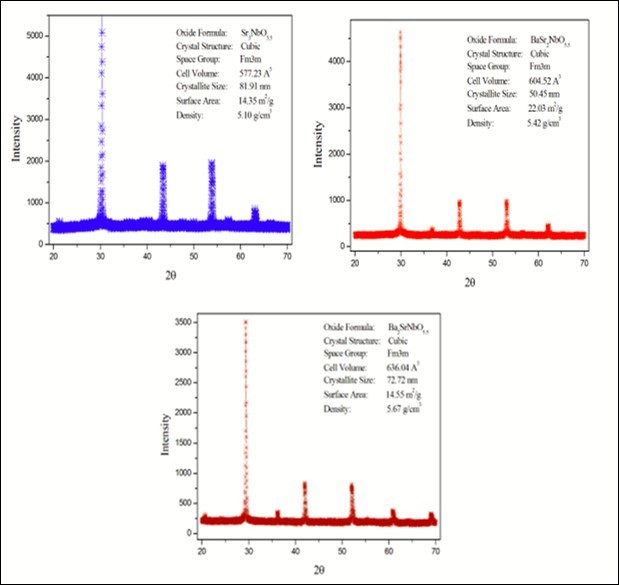The Average Crystallite size Dp, specific surface area S, lattice strain φ, Lattice parameter a and Cell volume V estimated from X-ray diffraction data are summarised in Table 1. The crystallite size can be calculated using sheerer formula13 (Equation. 1) where the specific surface area can be calculated using Sauter formula14 (Equation.2) in which ρ is the density of the synthesised material.

Table 1. Average Crystallite size Dp, Specfic surface area S, lattice strain φ, Lattice parameter a and Cell volume V. estimated from X-ray diffraction data.
 Formula D p ( ηm ) ρ(g/cm 3 ) S (m 2 /g) φ a (Å) V (Å3) Sr3NbO5.5 81.91 5.104 14.35 0.0028 8.3263(3) 577.230(1) BaSr2NbO5.5 50.45 5.419 22.03 0.0020 8.4554(2) 604.520(1) Ba2SrNbO5.5 72.72 5.669 14.55 0.0020 8.5999(3) 636.040(2)

Dp= (0.94λ)/(β1/2×cosϴ). …..(1)

S = 6000/ (Dp ×ρ). …..(2)

## Effect of Time

The removal percentage of dyes over the adsorbents can be calculated as: R% = ((Ci-Ct)/Ci) × 100, where R% is the removal percentage, Ci = 10 ppm is initial concentration of dye solution, Ct is the concentration of dye at contact time estimated from the concentration dependence of absorbance fit. Figure 2. shows the time dependence of MV removal at room temperature. There is no finite time was observed for the dye removal up to 150 min. The removals of the dye increase as the contact time increases. The removal of MV on the surface of Sr3NbO5.5, BaSr2NbO5.5 and Ba2SrNbO5.5 were found to be 66.15, 73.24 and 93.94% respectively. The removals of MV using Ba2SrNbO5.5 were larger than those using BaSr2NbO5.5 and Sr3NbO5.5. This is due to the effect of oxide composition. The increase in the organic dye removal is consisted with the increase in the Ba ion content.The inserted equations in Figure 2 describe the removal percentage (R%) as function of time (t) for eachoxide. The initial removal rate (dR/dt) could be derived from the equations when t=0. The initial removal rates for MV dye were found to be 3.25, 2.71 and 3.04 using Sr3NbO5.5, BaSr2NbO5.5 and Ba2SrNbO5.5 respectively. The wavelength dependence of absorbance for MV solution (Figure 3) illustrates the absorbance of MV solutions decreased as result of using BaxSr3-xNbO5.5 oxides as adsorbents. In addition, the absorbance decreases as the value of x in the formula increased.

Figure 2. the time dependence of MV removal at room temperature. The volume, concentration and pH of the dyes solution are 50ml, 10ppm and 5.1 respectively.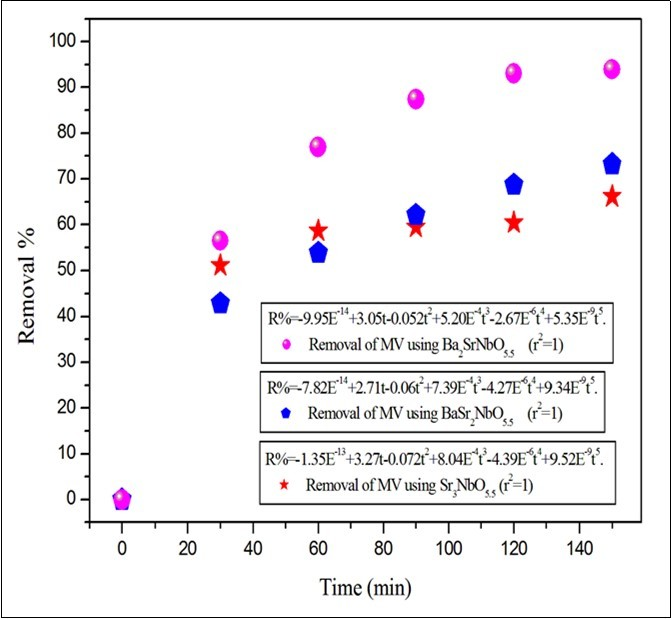Figure 3. The wavelength dependence of absorbance for MV solution. The time, volume, concentration, adsorbent mass and pH of MV solution are 150 min, 50ml, 10ppm, 0.1 g and 5.1 respectively.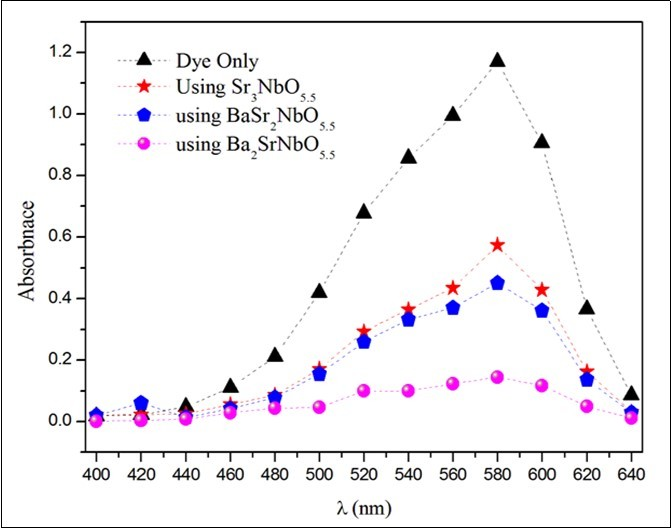The amount of the dye adsorbed by one gram of the oxides (Q) was calculated as following: Q (mg/g) = ((Ci-Ct)×V)/W, where t= 150min is the contact time, V= 50 ml is the volume of MV solution and W is the mass of oxides. As shown in Figure 4, Q decreases as the mass of adsorbents increased. The maximum capacity of adsorbent Qmax can be estimated from the intercept of the liner fit of 1/Qt at Y axis. Ba2SrNbO5.5 (72.72 nm, 14.55 m2/g) displayed the highest value of Qmax (46.47(2) mg/g) whereas Sr3NbO5.5 (81.91 nm, 14.35 m2/g) exhibited the lowest value of Qmax (8.03(5) mg/g). Qmax for BaSr2NbO5.5 (50.45 nm, 22.03 m2/g)is 13.09(2) mg/g. This result suggested an enhancement in the adsorption properties occurs as result of the substitution of Ba2+ into the oxide plus the decrease in crystallite size. The decrease in crystallite size leads to an increase in the surface area of particles.

Figure 4. The effect of adsorbent mass on the removal. The time, volume, concentration and pH of dye solutions are 150min, 50ml, 10ppm and 5.1 respectively.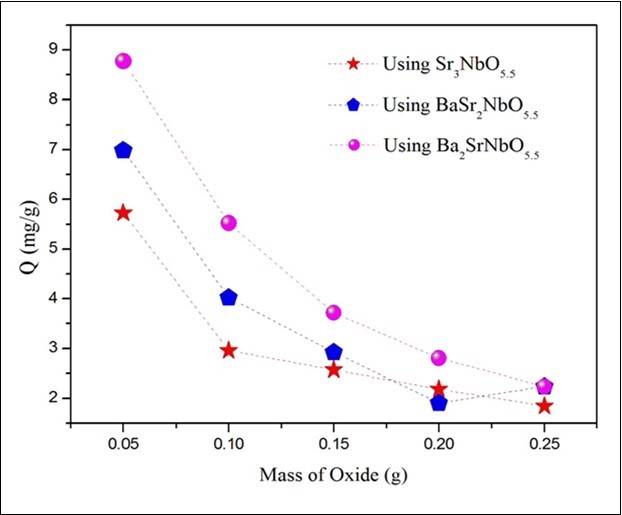## Effect of Temperature

Temperature has an important impact on the adsorption process. An increase in temperature helps the reaction to compete more efficiently with e/H+ recombination. The removal of two dyes was investigated at 25, 40, 60 and 100oC. The obtained results are illustrated below in Figure 5. The removal of MV dye increased as temperature increased. For instance, the removal of MV increased from ~64.9% at 25C to ~99% at 100C when BaSr2NbO5.5 was used. This result is agreed with normal expectations, and is a consequence of the increase of adsorption strength and the concentration of active intermediates with temperature. The energy of activation (Ea), was calculated from the Arrhenius plot of ln R vs 1000/T. Arrhenius plot shows that the activation energies of the removal are positive and equal to 4.79 and 4.32 kJ/mole for Sr3NbO5.5 and BaSr2NbO5.5 respectively. The activation energy of the removal was (-0.018 kJ/mole) for Ba2SrNbO5.5. This reflects the differences in the strength of the interaction forces between the dye and the oxides.

Figure 5. the effect of temperature on the MV removal. The time, volume, pH and concentration of dyes solutions are 150min, 50ml, 5.1 and 10ppm respectively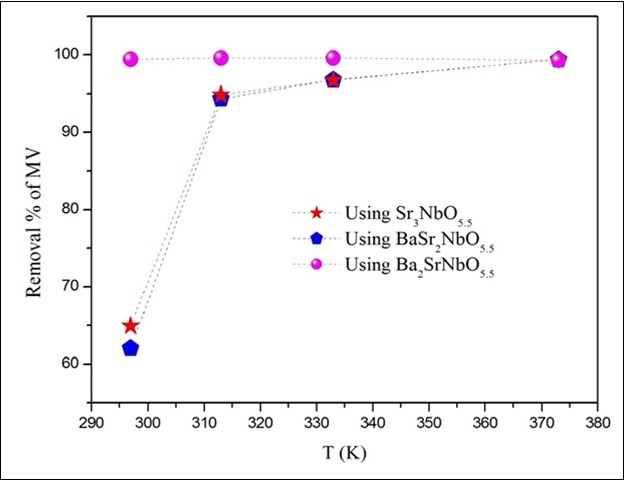## Effect of pH

The pH of solutions is a key parameter in dye adsorption. The magnitude of electrostatic charges which are impacted by the ionised dye molecules is controlled by the solution pH. As a result the rate of adsorption will vary with the pH of the medium used. In general, at low solution pH, the percentage of dye removal will decrease for cationic dye adsorption, while for anionic dyes the percentage of removal will increase. This is due to the increase in the positive charge on the solution interface and the adsorbent surface. In contrast, high solution pH is preferable for cationic dye adsorption but shows a lower efficiency for anionic dye adsorption. The positive charge at the solution interface will decrease while the adsorbent surface appears negatively charged.

To study the effect of pH, experiments were carried out at various pH values, ranging from 2 to 10 for constant dye concentration (10 ppm) and adsorbent mass (0.1g). Figure 6 presents the removal of dyes as a function of pH. It was observed that the removal of MV using the doped oxides increases as pH increased. The highest removal of MV was recorded at pH= 10 around 98.8 % using Ba2SrNbO5.5 where the lowest removal of MV was recorded at pH= 4 around 22% using the same oxide. The removal of MV using Sr3NbO5.5 has gradually increased from ~50% to ~75% as pH increased from 2 to 10.The removal of MV reached maximum (~91%) using BaSr2NbO5.5 at pH 2 and 10. The removal efficiency of the adsorbents is clearly increases as the acidity decreased.

Figure 6. the effect of pH on the removal of MV. The time, volume and concentration of dyes solution are 150min, 50ml and 10ppm respectively.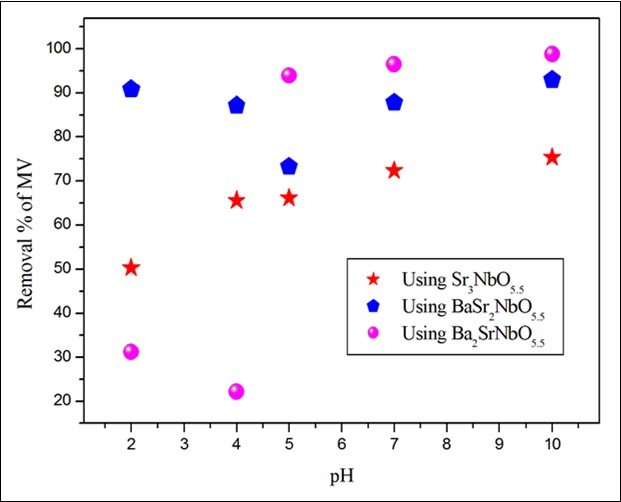## Conclusion

The removal of Methyl Violet from aqueous solution using the A- site doped perovskites BaxSr3-xNbO5.5 has been reported. The nano particle oxides were synthesised by solid state reaction and characterized by XRD. The results showed the substitution of Ba2+ has influenced both the texture and adsorption properties of the oxides. It was found that the adsorbent amounts of organic dye increases as the Ba2+ content increased. The removal of MV increases as the physical parameters: time, temperature, pH, adsorbent mass increased. The maximum capacities of adsorbent are 46.47, 13.09 and 8.03 mg/g for Ba2SrNbO5.5, BaSr2NbO5.5 and Sr3NbO5.5 respectively. The highest removal efficiency was recorded for MV dye using Ba2SrNbO5.5 at pH=10 where the lowest removal was observed for the same oxide at pH=2.